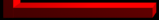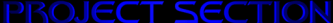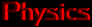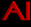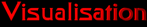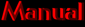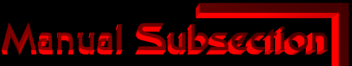This purpose of this section is to provide sufficent information on how to compose your own szenes and presentations in skeletronic.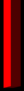With version 0.2b you get an easy to use GUI to select your stc source file, view its information and browse it. All previous files can still be viewed with the new stcbrowser ( they might look a little diffrent ) All the information about a szene is stored in a single textfile with the extension .stc .The file is divided into different units like worlds, vertices, models, textures, lights, camerapaths and the presentation.
StcInfo ( The Information here can be previewed with the GUI )
stcinfo {
}
Start the Infoblock
title = [titlename]; The name of your Presentaion
author = [authorname]; The guy who made the scene
desription = [text]; A short summery of what the file contains or whatever you want to tell the world
version = [browserdll]; The libraryversion required to view the file. ( Default is stcbrowser.dll )
Worlds ( here goes all the data which physics will be applied to )
world_begin
world_end
Encloses a worlds unit. ( There can be multiple ones )
name = [worldname]; Identifies this world for later use. Each world must have a unique name
object_begin
object_end
Encloses an object subunit ( they receive names as well )
vertex { . . .
}
Masspoints are defined here
name = [vertexname]; vertices dont need individual names. They can be identified by their index. So vertexname is the fourth vertex.
weight = #; Set the weight for the following masspoints. Use -1 for fixed points
vector = ( x, y, z ); new masspoint with coordinates x, y, z
}
Connections for the masspoints
elasticity = #; Change the elasticity for the following links
friction= #; Change friction
k = #; Deprecated use elasticity
f = #; Deprecated use friction
length = #; Different standardlength for next link
triangles { . . .
}
Planes go in here
friction = #; Friction for moving objects
stickyness = #; Friction for nonmoving objects
k0 = #; Deprecated use friction
k1 = #; Deprecated use stickyness
triangle = ( p1, p2, p3 ); Triangle from points p1, p2 and p3
gen_tuch { . . .
}
Generates a grid of physic points.
Commands like weight, friction etc work here as well.
name = #; The name of the grid
center = ( x, y, z ); The location of the point in the center
steps = ( x, y, 0 ); How many steps ( tiles )
stepx = ( x, y, z ); The first vector seperating the points.
stepy = ( x, y, z ); The second vector seperating the points.
linecolor = ( r, g, b, a ); Defines the linecolor. ( values range from 0 to 1. the alpha value is used for opacity )
trianglecolor = ( r, g, b, a ); Defines the trianglecolor
vertexcolor = ( r, g, b, a ); Defines the vertexcolor
matrix{ . . .
}
Used to translate, rotate and scale your world
identity; Create a new 4 by 4 identity matrix
rotate = ( a, x, y, z ); Rotate the coordinatesystem by a degrees in mathmatical positive direction. Using the vector ( x, y, z ).
translate = ( x, y, z ); Translate the coordinatesystem by ( x, y, z )
vertex { . . .
}
Calculated vertices
name = vertexname; Name of the following vertices. Indexing works here too
average = expr; expr = ( faktor*anyvertex + ... ) + raising
Static vertices ( these wont pass through physics )
vertex { . . .
}
Encloses a vertex unit
name = vertexname; Name of following vertices
static = ( x, y, z ); add new vertex
precache = #; with precaching you announce another n vertices will get inserted (faster loading)
Animated vertices ( used for visualisation only )
moving { . . .
}
Encloses a movingvertex unit
name = vertexname; Name of following splines
loops = #; Number of cycles. Use -1 for an endless loop
spline { . . .
}
Start new splinepath
dt = #; Time intervall
vector = ( x, y, z ); New splinecorner
anyvertex - calling previously declared vertices
Physical points worldname.objectname.vertexname[index]
Static vertices static.vertexname[index]
Calculated vertices calc.vertexname[index]
Animated vertices moving.vertexname[index]
Textures
textures { . . .
}
encloses a texture unit
name = texturename; You can index textures like vertices
texturemode = mode; Decide whether you want to use textures or mipmaps or both. Textures are faster. But Trilinear filtering looks better. Possible values for texturemode are nearest (faster) and linear (more detail)
mipmapmode = mode; Possible values : nearest_nearest ( fastest ), nearest_linear, linear_nearest and linear_linear ( most detail )
texture = filename; Add a texture. Use relative filenames. When you pass this file to the stcbrowser the path must be correct or your textures wont load.
mipmap = filename; Add a mipmap. They use approximatly 1.7 times the space textures do on your graphiccard. For textures and mipmaps jpeg images are used. The size should be 2m*2n
Lighting
gllights { . . .
}
Define Lights
name = lightname; Light ids
spotexp, cutoff, const_att, linear_att, quad_att = #; Properties for the light. ( But you can leave the preselection if you want )
ambient, diffuse, specular = ( r, g, b, a ); The lightcolor
direction = ( x, y, z ); or destination = anyvertex; Determines where the light goes.
position = ( x, y, z, w ); or origin = anyvertex; Both commands define the position of the light, once as an allready defined vertex and once as a vector. These instructions will add the light. So define the other properties befor calling position or origin.
Models
model_begin
model_end
Like worlds but there is no physical calculation
name = modelname; Each model has a unique name and must receive an extra block
color = ( r, g, b, a ); Assigns the color
texselect = texturename[index]; Picks the texture.
polygon, fan, strip { . . .
};
Encloses a polygon subunit. Fan and Strip determins the order in which the plots are drawn
color = ( r, g, b, a ); Changes the color.
texcoord = ( x, y ); Assign the texturecoordinate for the next plot.
plot = anyvertex; Draws a new plot
cone { . . .
};
Draws cones, cylinders and circles
r1, r2, height = #; Radius 1, 2 and the height. If one radius is zero it's a cone. If both are equal its a cylinder.
tex_x, tex_y = #; Texture tiles.
mode = conemode; Determins which parts of the cone are actually drawn. Possible values: full, top, bottom, side, top_and_bottom, side_and_top, side_and_bottom.
matrix = ( a11, a12, . . ., a44 ); This way you define where the cone gets displayed. ( You can enter rotation, position and scaling in the matrix Other effects are also possible ) - I know the cone declaration is kinda complicated. I will change that with the next release.
sphere { . . .
}
Draws spheres and elipsoids
a, b = #; Of a and b are equal the object will turn out to be a sphere. Otherwise it's an elipsoid.
matrix = ( a11, a12, . . ., a44 ); Works like the cones.
mesh { . . .
}
Draw a mesh of points
tiles = ( x, y ); How many section the grid gets divided into.
type = [color/texcoord/vertex]( x, y ); Specify what kind of values follow and how many of them. so type = texcoord( 2, 2 ) means 4 texcoord entries.
values = #; Supply the values for the selected type. ( ( r, g, b, a ) for color; ( x, y ) for texcoord and a vertexreference for vertices.
precache = #; amount of instructions
Rendering
render_begin
render_end
Encloses a render unit
name = rendername; Each redner unit has a unique name and must receive an extra block
enable, disable = value; Enables and disables certain modes. Possible values are: texture, wireframe, blend, zbuffer, light0-7 (only 8 lights may be used at once), alllight, cullfront, cullback, lighting and znotest
clear = value; values are screen, zbuffer and zflip
gllight = 0-7, lightname; choose which lights to use
model = modelname; Render a model
world = worldname; Render a world
effects_init { . . .
};
Effects initialisation ( the old command shadow_init {} and shadow_done stills works )
direction = ( x, y, z ); Parallel Light ( like sunlight ). This instruction goes together with parallel = ;
origin = anyvertex; Light Position
destination = ( p1, p2 ); Where does the light show
perspective = ( p1, p2, p3, p4 ); Perspective shadow to polygon
parallel = ( p1, p2, p3, p4 ); Parallel shadow to polygon
enable = reflextion; Produces a reflextion on the z = 0 plane
model = [modelname]; You can supply you own model to draw the shadow/reflextion plane.
effects_done; Every object between effects_init and effects_done casts shadow or reflextions
Cameraviews
camera_begin
camera_end
Encloses a camera unit
name = cameraname; Camera id.
lookat_vertex, camera_vertex = anyvertex; Camera location and the vertex which the camera points on.
distance_value, roll_value = #; Distance defines how the projection is handled. And a roll value of 180 for Example turnes the world upside down.
lookat_path, camera_path { . . .
}
camera_path defines a splinepath for the cameraposition. lookat_path is a splinepath for the way the camera points to.
dt = #; Time intervall
vector = ( x, y, z ); New corner
vertex = anyvertex; New corner from a defined vertex
distance_path, roll_path { . . .
}
with distance_path you can create zooming effects. roll_path dynamically changes the rollvalue.
dt = #; Time intervall
value = #; New distance or rollvalue.
render = rendername, time; Assigns a renderer to the camera and the time before reset
reset; Resets the camera.
Presentation (main)
presentation_begin
presentation_end
Encloses the presentation unit. ( There is only one. And it is declared after everything else )
wait = #; Wait for #ms and update phyisc, screen and spiles
mode = value; Modes are full and split4
opengl_window = ( w, h, x, y ); Creates an OpenGL window with width = w, height = h at position x, y. ( If x and y are off screen the window will be centered )
opengl_full = ( w, h ); Creates an OpenGL window in fullscreenmode.
marke, loop = label; Marke sets the label and loop jumps there.
window { . . .
}
creates a moving window
camera = cameraname; Assigns the camera.
p0, p1 = anyvertex; Upperleft and lowerright corner of the window
set = #; Sets the screennumber
camera = cameraname, screenpart_no; Which camera for which screenpart. ( In fullmode the screenpart is 0, in split4 1-4 )
physikstep = steps_per_second; How much detaik for physics.
update = worldname; Updates a specific world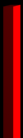All right that's it. Now you can become a perfect skeletronic designer. Feel free to send me your work. I will publish it on this page.